# 4 1.3 Converting Units

It is sometimes important to convert between different types of units. Let us consider a simple example of how to convert units. Let us say that we want to convert 80 meters (m) to kilometers (km).

The first thing to do is to list the units that you have and the units that you want to convert to. In this case, we have units in meters and we want to convert to kilometers.

Next, we need to determine a conversion factor relating meters to kilometers. A conversion factor is a ratio expressing how many of one unit are equal to another unit. For example, there are 100 centimeters in 1 meter, 60 seconds in 1 minute, and so on. In this case, we know that there are 1,000 meters in 1 kilometer.

Now we can set up our unit conversion. We will write the units that we have and then multiply them by the conversion factor so that the units cancel out, as shown:

$\bf{80 \;\rule[0.5ex]{1.0em}{0.1ex}\hspace{-1.0em}\textbf{m}\times}$$\bf{\frac{1\textbf{ km}}{1000\;\rule[0.25ex]{0.75em}{0.1ex}\hspace{-0.75em}\textbf{m}}}$$\bf{= 0.080\textbf{ km}}$

Note that the unwanted m unit cancels, leaving only the desired km unit. You can use this method to convert between any types of unit.

Here’s another way to think about it:

Consider a simple example: how many cm are there in 4 meters? You may simply think there are 400cm in 4 meters. How did you make this determination? Well, if there are 100 cm in 1 m and there are 4 meters, then there are 4 × 100 = 400cm in 4 meters.

This is correct, of course, but it is informal. Let us formalize it in a way that can be applied more generally. We know that 1 m equals 100 cm:

1 m = 100 cm

In math, this expression is called an equality. The rules of algebra say that you can change (i.e., multiply or divide or add or subtract) the equality (as long as you don’t divide by zero) and the new expression will still be an equality. For example, if we divide both sides by 2, we get

1/2 m = 100/2 cm

We see that one-half of a meter equals 100/2, or fifty cm—something we also know to be true, so the above equation is still an equality. Going back to the original equality, suppose we divide both sides of the equation by 1 meter (number and unit):

1/1m = 100cm/1m

The expression is still an equality, by the rules of algebra. The left fraction equals 1. It has the same quantity in the numerator and the denominator, so it must equal 1. The quantities in the numerator and denominator cancel, both the number and the unit. When everything cancels in a fraction, the fraction reduces to 1:

1 = 100cm/1m

We have an expression, 100 cm / 1m, that equals 1. This is a strange way to write 1, but it makes sense: 100 cm equal 1 m, so the quantities in the numerator and denominator are the same quantity, just expressed with different units. The expression 100 cm / 1m is called a conversion factor, and it is used to formally change the unit of a quantity into another unit. (The process of converting units in such a formal fashion is sometimes called dimensional analysis or the factor label method.)

To see how this happens, let us start with the original quantity:

4 m

Now let us multiply this quantity by 1. When you multiply anything by 1, you don’t change the value of the quantity. Rather than multiplying by just 1, let us write 1 as 100cm/1m:

4 m X 100cm/1m

The 4 m term can be thought of as 400cm/1; that is, it can be thought of as a fraction with 1 in the denominator. We are essentially multiplying fractions. If the same thing appears in the numerator and denominator of a fraction, they cancel. In this case, what cancels is the unit meter:

400cm

That is all that we can cancel. Now, multiply and divide all the numbers to get the final answer:

Again, we get an answer of 400 cm, just as we did originally. But in this case, we used a more formal procedure that is applicable to a variety of problems.

Want more examples?

How many millimeters are in 14.66 m? To answer this, we need to construct a conversion factor between millimeters and meters and apply it correctly to the original quantity. We start with the definition of a millimeter, which is

1 mm = 1/1,000 m

The 1/1,000 is what the prefix milli- means. Most people are more comfortable working without fractions, so we will rewrite this equation by bringing the 1,000 into the numerator of the other side of the equation:

1,000 mm = 1 m

Now we construct a conversion factor by dividing one quantity into both sides. But now a question arises: which quantity do we divide by? It turns out that we have two choices, and the two choices will give us different conversion factors, both of which equal 1: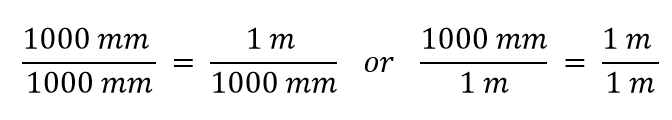Which conversion factor do we use? The answer is based on what unit you want to get rid of in your initial quantity. The original unit of our quantity is meters, which we want to convert to millimeters. Because the original unit is assumed to be in the numerator, to get rid of it, we want the meter unit in the denominator; then they will cancel. Therefore, we will use the second conversion factor. Canceling units and performing the mathematics, we get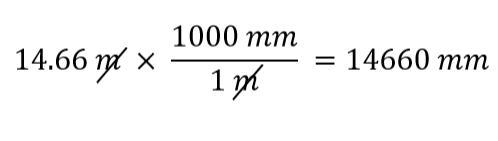Note how m cancels, leaving mm, which is the unit of interest.

The ability to construct and apply proper conversion factors is a very powerful mathematical technique in biomechanics. You need to master this technique if you are going to be successful in this and future courses.

### Applied Example: Unit Conversions: A Short Drive Home

Suppose that you drive the 10.0 km from your university to home in 20.0 min. Calculate your average speed (a) in kilometers per hour (km/h) and (b) in meters per second (m/s). (Note: Average speed is distance traveled divided by time of travel.)

Strategy

First we calculate the average speed using the given units. Then we can get the average speed into the desired units by picking the correct conversion factor and multiplying by it. The correct conversion factor is the one that cancels the unwanted unit and leaves the desired unit in its place.

Solution for (a)

(1) Calculate average speed. Average speed is distance traveled divided by time of travel. (Take this definition as a given for now—average speed and other motion concepts will be covered in a later module.) In equation form,

$\bf{\textbf{average speed}=}$$\bf{\frac {\textbf{distance}}{\textbf{time}}}$.

(2) Substitute the given values for distance and time.

$\bf{\textbf{average speed} =}$$\bf{\frac {10.0\textbf{ km}} {20.0\textbf{ min}}}$$\bf{=0.500}$$\bf{\frac {\textbf{ km}} {\textbf{ min}}}$

(3) Convert km/min to km/h: multiply by the conversion factor that will cancel minutes and leave hours. That conversion factor is 60 min/hr. Thus,

$\bf{\textbf{average speed}=0.500}$$\frac{\textbf{km}}{\textbf{min}}$$\bf{\times}$$\bf{\frac{60\textbf{ min}}{1\textbf{ h}}}$$\bf{=30.0}$$\bf{\frac{\textbf{km}}{\textbf{h}}}$.

Discussion for (a)

(1) Be sure that you have properly cancelled the units in the unit conversion. If you have written the unit conversion factor upside down, the units will not cancel properly in the equation. If you accidentally get the ratio upside down, then the units will not cancel; rather, they will give you the wrong units as follows:

$\bf{\frac{km}{min}}$$\bf{\times}$$\bf{\frac{1\textbf{ hr}}{60\textbf{ min}}}$$\bf{=}$$\bf{\frac{1\textbf{ km}\cdot\textbf{hr}}{60\textbf{ min}^2}}$,

which are obviously not the desired units of km/h.

(2) Check that the units of the final answer are the desired units. The problem asked us to solve for average speed in units of km/h and we have indeed obtained these units.

(3) Round to two decimal places. The answer can be left as 30 km/h since it is a whole value.

(4) Next, check whether the answer is reasonable. Let us consider some information from the problem—if you travel 10 km in a third of an hour (20 min), you would travel three times that far in an hour. The answer does seem reasonable.

Solution for (b)

There are several ways to convert the average speed into meters per second.

(1) Start with the answer to (a) and convert km/h to m/s. Two conversion factors are needed—one to convert hours to seconds, and another to convert kilometers to meters.

(2) Multiplying by these yields

$\bf{\textbf{Average speed}=30.0}$$\bf{\frac{km}{h}}$$\bf{\times}$$\bf{\frac{1\textbf{ h}}{3,600\textbf{ s}}}$$\bf{\times}$$\bf{\frac{1,000\textbf{ m}} {1\textbf{ km}}}$,
$\bf{\textbf{Average speed} = 8.33}$$\bf{ \frac {m} {s}}$.

Discussion for (b)

If we had started with 0.500 km/min, we would have needed different conversion factors, but the answer would have been the same: 8.33 m/s.

What if we have a derived unit that is the product of more than one unit, such as m2? Suppose we want to convert square meters to square centimeters? The key is to remember that m2 means m × m, which means we have two meter units in our derived unit. That means we have to include two conversion factors, one for each unit. For example, to convert 17.6 m2 to square centimeters, we perform the conversion as follows: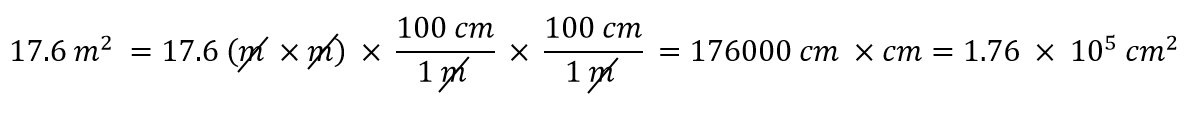### Practice Problem

How many cubic centimeters are in 0.883 m3?

Solution

With an exponent of 3, we have three length units, so by extension we need to use three conversion factors between meters and centimeters. Thus, we have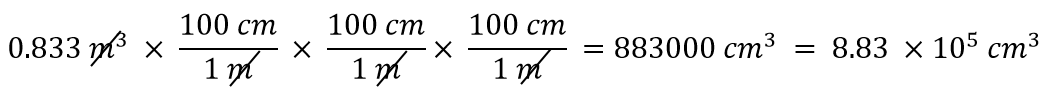You should demonstrate to yourself that the three meter units do indeed cancel.

Suppose the unit you want to convert is in the denominator of a derived unit; what then? Then, in the conversion factor, the unit you want to remove must be in the numerator. This will cancel with the original unit in the denominator and introduce a new unit in the denominator. The following example illustrates this situation.

### Practice Problem

Convert 88.4 m/min to meters/second.

Solution

We want to change the unit in the denominator from minutes to seconds. Because there are 60 seconds in 1 minute (60 s = 1 min), we construct a conversion factor so that the unit we want to remove, minutes, is in the numerator: 1 min/60 s. Apply and perform the math: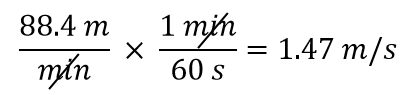Notice how the 88.4 automatically goes in the numerator. That’s because any number can be thought of as being in the numerator of a fraction divided by 1.

Sometimes there will be a need to convert from one unit with one numerical prefix to another unit with a different numerical prefix. How do we handle those conversions? Well, you could memorize the conversion factors that interrelate all numerical prefixes. Or you can go the easier route: first convert the quantity to the base unit, the unit with no numerical prefix, using the definition of the original prefix. Then convert the quantity in the base unit to the desired unit using the definition of the second prefix. You can do the conversion in two separate steps or as one long algebraic step. For example, to convert 2.77 kg to milligrams:

Alternatively, it can be done in a single multistep process: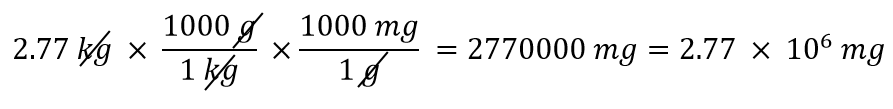You get the same answer either way.

How do I round my final answer?

For the purpose of this class, you can round your answer to two decimal places. For example, if your final answer of the force exerted during an Olympic deadlift is 783.4723 N, you can round to 783.47 N.

The third number after the decimal will decide how to proceed:

1. If the number you are rounding is followed by 5, 6, 7, 8, or 9, round the number up. Example: 38.345 will round to 38.35.
2. If the number you are rounding is followed by 0, 1, 2, 3, or 4, round the number down. Example: 38.342 will round to 38.34.
3. If the final answer is a whole number: 38.00000 you can write your answer as 38.

### Key Takeaways

• Units can be converted to other units using the proper conversion factors.
• Conversion factors are constructed from equalities that relate two different units.
• Conversions can be a single step or multistep.
• Unit conversion is a powerful mathematical technique in biomechanics that must be mastered.
• In this course, you can round your answer to two decimal places.